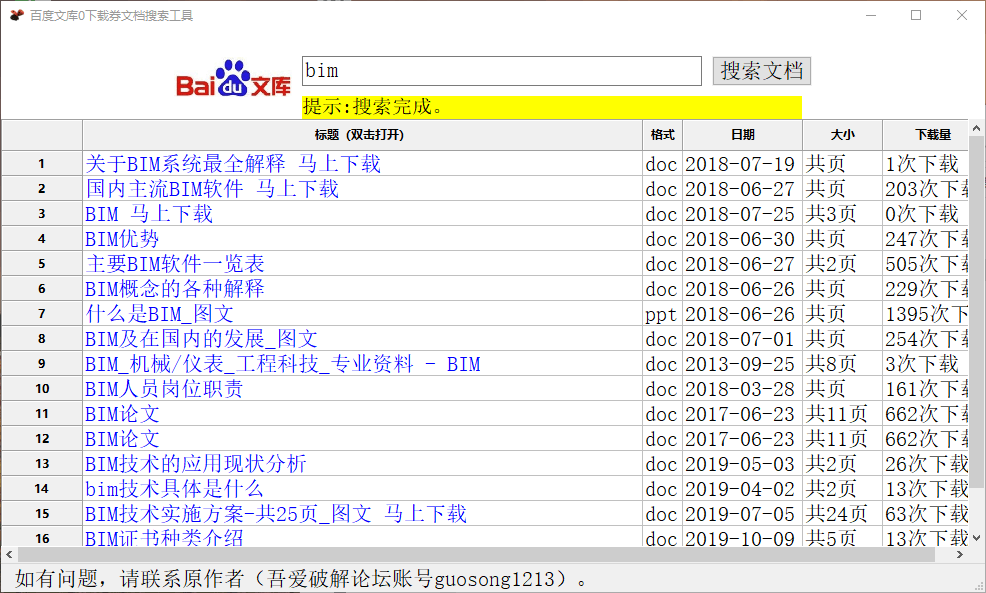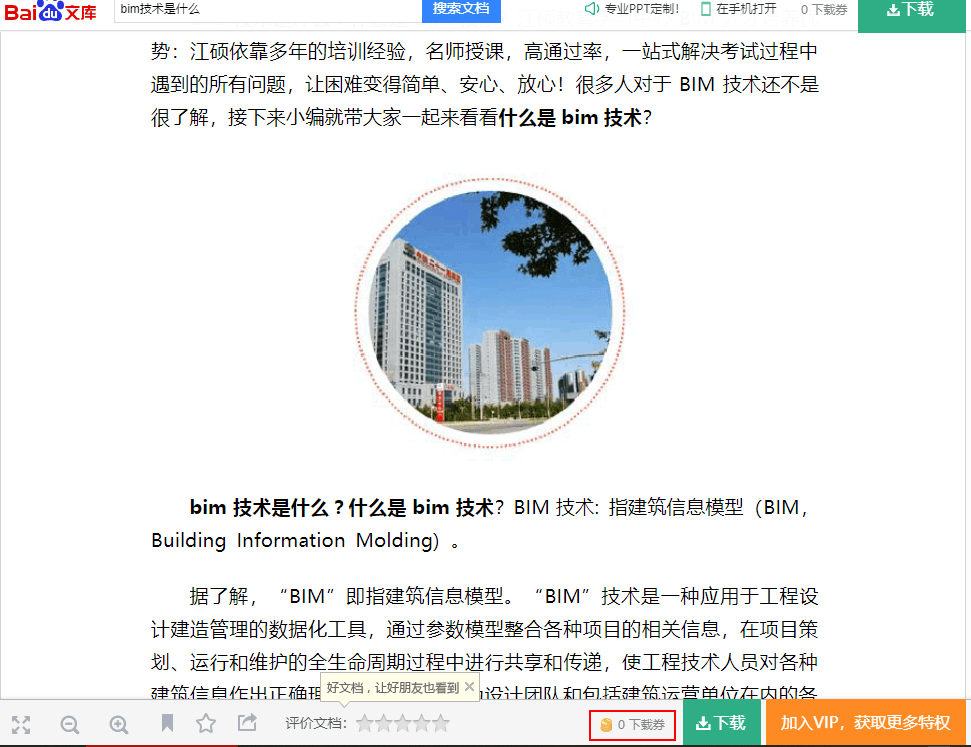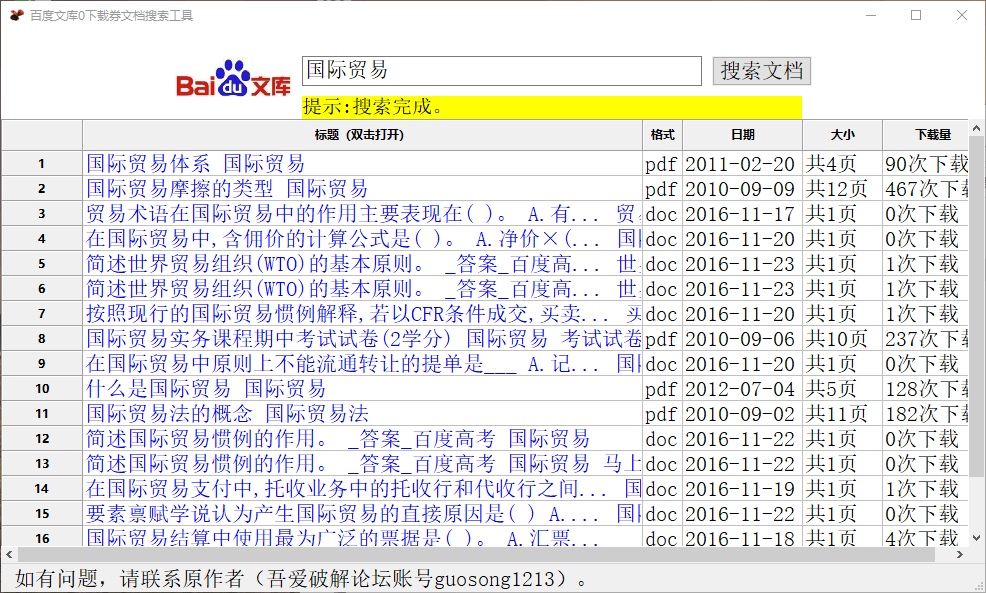# 百度文库0下载券文档搜索工具

`from urllib import parse`
`import requests`
`import webbrowser`
`from pyquery import PyQuery as pq`

`def getPage(keyW):`
`pn = 0#从第1页开始`
`try:`
`while keyW != '':#关键词不能为空`
`url = 'https://wenku.baidu.com/search?word=' + keyW + '&org=0&fd=0&od=0&pn=' + str(pn)`
`r = requests.get(url)`
`r.encoding = 'gb2312'#需要先将获取的源码进行编码再交给pyquery，否则汉字都是乱码`
`doc = pq(r.text)`
`len1 = len(doc('dl'))#dl是每条搜索内容的标签，这一句是获取当前页的搜索数`
`if pn == 0 and len1 == 0:`
`#百度文库中没有搜索不到结果的情况。如果第一页就没有结果，说明需要验证网页，`
`# 因为如果搜索太频繁，百度文库会提示需要验证`
`print("提示:因操作频繁，本次需要验证。验证完成后，重新搜索。")`
`webbrowser.open(url, new=0)#这一句可以直接打开浏览器，自己手动在网页上验证。`
`# 验证完跳出循环，运行结束。自己再重新执运行码。每天最多只需验证一次。`
`break`
`else:`
`for dl in range(len1):#遍历所有结果`
`list = []`
`dl_eq = doc('dl').eq(dl)#这样获取的dl标签的源码，这一句很重要`
`if 'VIP专享' not in dl_eq.find('.ico-vip-special').text() and '0下载券' in dl_eq.find('.detail-info').text():`
`#判断是不是0下载券，又没有VIP专享`
`list.append(dl_eq.find('.log-xsend').text())#标题写入list数组`
`list.append(dl_eq.find('.ic').attr('title'))#格式写入list数组`
`list.append(dl_eq.find('.detail-info').text().split('|', 4))#日期写入list数组`
`list.append(dl_eq.find('.detail-info').text().split('|', 4))#大小写入list数组`
`list.append(dl_eq.find('.detail-info').text().split('|', 4))#下载量写入list数组`
`list.append(dl_eq.find('.log-xsend').attr('href'))#链接写入list数组`
`print(list)`
`if len(doc('.page-content .next'))==0:#当网页中没有下一页标签就是最后一页了。.page-content .next是下一页的class`
`print("提示:搜索完成。")`
`break`
`pn = pn+10#进入下一页`
`else:`
`print("提示:请输入关键词。")`
`except requests.exceptions.ConnectionError:`
`print("提示:网络异常，请检查网络并重新搜索！")`

`if __name__ == '__main__':`
`keyWord = "三生三世十里桃花".replace(' ', '')#去掉关键词中的空格，关键词根据个人需要修改`
`keyW = parse.quote(keyWord.encode('gb2312'))#对关键词进行编码`
`getPage(keyW)#需要搜索的关键词`